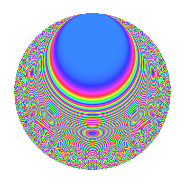# Properties

 Label 1001.2.bLevel 1001 Weight 2 Character orbit b Rep. character $$\chi_{1001}(846,\cdot)$$ Character field $$\Q$$ Dimension 96 Newform subspaces 2 Sturm bound 224 Trace bound 6

# Related objects

## Defining parameters

 Level: $$N$$ = $$1001 = 7 \cdot 11 \cdot 13$$ Weight: $$k$$ = $$2$$ Character orbit: $$[\chi]$$ = 1001.b (of order $$2$$ and degree $$1$$) Character conductor: $$\operatorname{cond}(\chi)$$ = $$77$$ Character field: $$\Q$$ Newform subspaces: $$2$$ Sturm bound: $$224$$ Trace bound: $$6$$

## Dimensions

The following table gives the dimensions of various subspaces of $$M_{2}(1001, [\chi])$$.

Total New Old
Modular forms 116 96 20
Cusp forms 108 96 12
Eisenstein series 8 0 8

## Trace form

 $$96q - 92q^{4} - 96q^{9} + O(q^{10})$$ $$96q - 92q^{4} - 96q^{9} + 8q^{11} + 4q^{14} - 16q^{15} + 108q^{16} - 12q^{22} + 48q^{23} - 120q^{25} + 96q^{36} + 48q^{37} - 66q^{42} + 36q^{44} + 60q^{49} - 78q^{56} - 64q^{58} - 64q^{60} + 4q^{64} - 32q^{67} + 36q^{70} - 8q^{71} - 4q^{77} + 20q^{78} + 128q^{81} - 32q^{86} + 12q^{88} - 8q^{91} - 204q^{92} + 8q^{93} - 92q^{99} + O(q^{100})$$

## Decomposition of $$S_{2}^{\mathrm{new}}(1001, [\chi])$$ into newform subspaces

Label Dim. $$A$$ Field CM Traces $q$-expansion
$$a_2$$ $$a_3$$ $$a_5$$ $$a_7$$
1001.2.b.a $$48$$ $$7.993$$ None $$0$$ $$0$$ $$0$$ $$4$$
1001.2.b.b $$48$$ $$7.993$$ None $$0$$ $$0$$ $$0$$ $$-4$$

## Decomposition of $$S_{2}^{\mathrm{old}}(1001, [\chi])$$ into lower level spaces

$$S_{2}^{\mathrm{old}}(1001, [\chi]) \cong$$ $$S_{2}^{\mathrm{new}}(77, [\chi])$$$$^{\oplus 2}$$

## Hecke Characteristic Polynomials

There are no characteristic polynomials of Hecke operators in the database